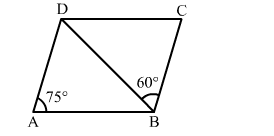# In the given figure, ABCD is a parallelogram in which ∠BAD = 75° and ∠CBD = 60°.

Question:

In the given figure, ABCD is a parallelogram in which BAD = 75° and ∠CBD = 60°. Then, ∠BDC = ?
(a) 60°
(b) 75°
(c) 45°
(d) 50°Solution:

(c) 45°

Explanation:
∠B = 180o − ∠A
⇒ ∠B = 180o − 75o = 105o
Now, ∠B =​ ∠ABD + ∠CBD
⇒​​ 105o​ = ∠ABD + 60o
⇒ ∠ABD​ = 105o − 60o = 45o
⇒ ∠ABD = ​∠BDC​ = 45         (Alternate angles)